Finally, a Math Education Tool that Supports Research-Based Learning Theory!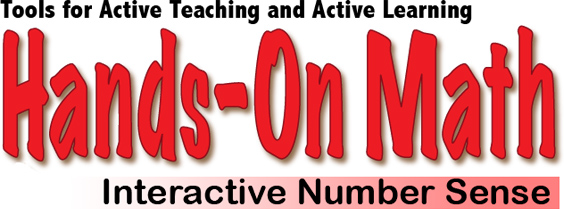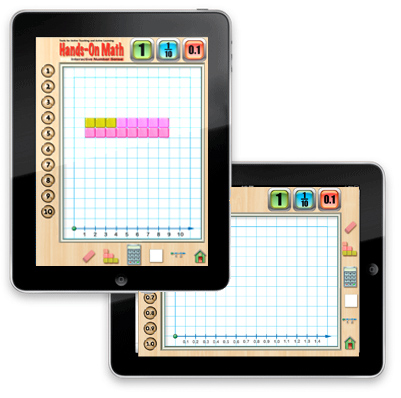Hands-On Math: Interactive Number Sense creates a virtual math playground where students explore, investigate and discover mathematical concepts. When students are interacting with the Number Sense Playground colored number bars are used to represent whole numbers, fractions and decimals. Using Hands-On Math: Interactive Number Sense students can develop the following important mathematical concepts: Ordering and comparing whole numbers, decimals and fractions. Adding, subtracting, multiplying and dividing whole numbers, fractions and decimals. Locating whole numbers, fractions or decimals on a number line.We recommend that you open the Instructor's Guide PDF from your iPad in a new browser page and save the document in your iBooks app.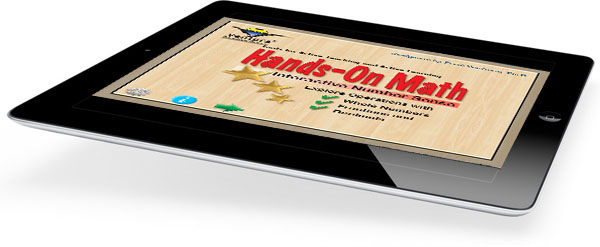Features: Open-ended Interactive Number Bars Playground. Supports Discovery and Directed Teaching Strategies . Number Pad Randomly Generates Quiz Questions which Can Be Solved Using Virtual Manipulatives. Number Bars Represent Whole Numbers, Fractions and Decimals On/Off Options and Controls for Various Features. \$2.99
 Number Bars Playground - Whole Numbers Colorful Number Bars are used to represent all the numbers from 1 to 10. The playground offers ample room for the Number Bars to be moved, arranged, and grouped in order to represent mathematical ideas. Topics: Compare and Order Whole Numbers Basic Operations of Add, Subtract, Multiply and Divide Properties of Real Numbers, for Example - The Commutative Property Locating Numbers on a Number Line Fact Families Greater Than, Less Than and Equal To Problem Solving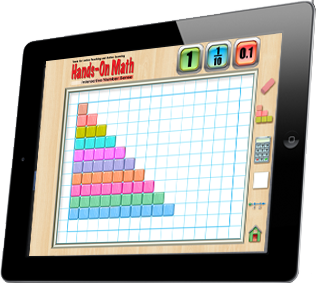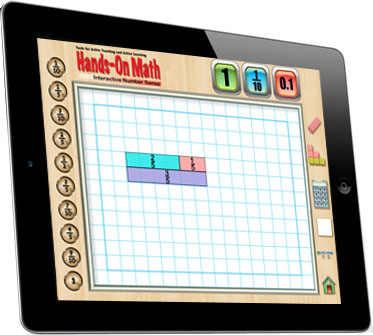Number Bars Playground - Fractions In Fraction Mode, the bars on Number Bars Playground are labeled with the fractions 1/10, 1/5, 3/10, 2/5, 1/2, 3/5, 7/10, 4/5, 9/10 and 1: Compare and Order Fractions Basic Operations with Fractions Least Common Multiple (LCM) Greatest Common Factor (GCF) Number Bars Playground - Decimals In Decimal Mode, the bars on Number Bars Playground are labeled with the decimals 0.1, 0.2, 0.3, 0.4, 0.5, 0.6, 0.7, 0.8, 0.9, 2.0: Compare and Order Fractions and Decimals Basic Operations with Decimals Locate Decimals on a Number Line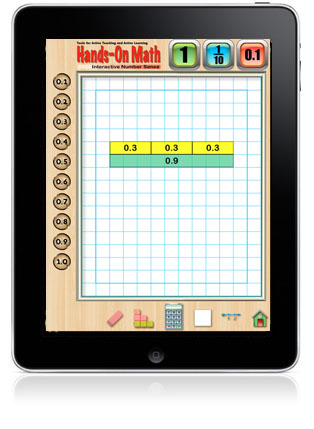Number Pad Quiz The Number Pad Quiz generates random addition problems to solve using using the Number Bars. Students tap the key pad to enter answers after using the Number Bars to investigate the problem. Problems are presented that involve whole numbers, fractions and decimals.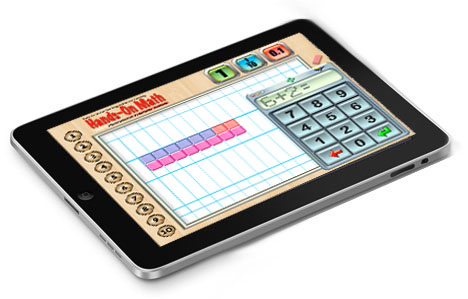Hands-On Math: Interactive Number Sense is now available at the Apple iTunes Store.